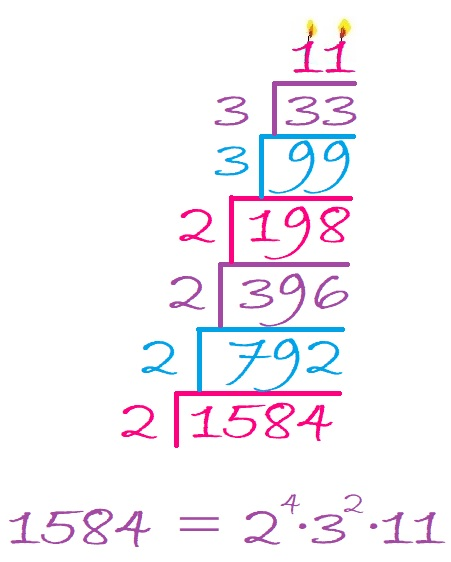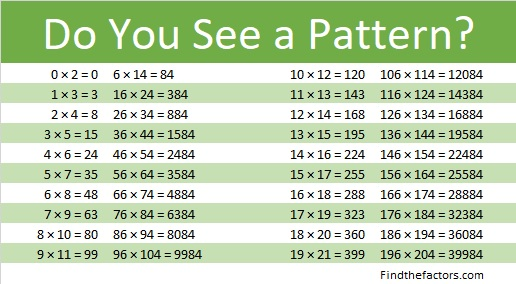# 1584 You Will L♥ve This Challenge Puzzle!

### Today’s Puzzle:

Here’s a Valentine’s challenge for you: Can you write the numbers from 1 to 10 in each of the four areas of the puzzle that touch the box with the X so that the numbers you write make the puzzle become four multiplication tables? It won’t be easy, but remember to use logic. If you succeed, you will absolutely lve the puzzle!### 1584 Factor Cake:

1584 obviously is divisible by 2. It is divisible by 4 because 84 is divisible by 4.
It is divisible by 8 because 84 is not divisible by 8 and 5 is odd.
1584 is divisible by 3 and by 9 because 1 + 5 + 8 + 4 = 18, a number divisible by 3 and by 9.
1584 is divisible by 11 because 1 – 5 + 8 – 4 = 0.

Thus, 1584 makes a very tall and very nice factor cake with a couple of candles on top!### Factors of 1584:

• 1584 is a composite number.
• Prime factorization: 1584 = 2 × 2 × 2 × 2 × 3 × 3 × 11, which can be written 1584 = 2⁴ × 3² × 11.
• 1584 has at least one exponent greater than 1 in its prime factorization so √1584 can be simplified. Taking the factor pair from the factor pair table below with the largest square number factor, we get √1584 = (√144)(√11) = 12√11.
• The exponents in the prime factorization are 4, 2 and 1. Adding one to each exponent and multiplying we get (4 + 1)(2 + 1)(1 + 1) = 5 × 3 × 2 = 30. Therefore 1584 has exactly 30 factors.
• The factors of 1584 are outlined with their factor pair partners in the graphic below.### More about the Number 1584:

1584 is the difference of two squares NINE different ways:
397² – 395² = 1584,
200² – 196² = 1584,
135² – 129² = 1584,
103² – 95² = 1584,
72² – 60² = 1584,
53² – 35² = 1584,
47² – 25² = 1584,
45² – 21² = 1584, and
40² – 4² = 1584.
Yes, we are only 16 numbers away from 1600, the next perfect square!

1584 is in this cool pattern: